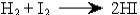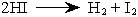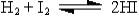MainTutorialsOrganic ChemistryPractice TestsOnline QuizzesReference ToolsChemical Equilibrium

What is Chemical Equilibrium?
As the name equilibrium suggests, it is some kind of balance. In this case it is a chemical rate balance.

chemical equilibrium = occurs when opposing reactions are going at the same rate.

This concept is actually very simple. Let's look at an example of a chemical equilibrium.If we put some H and I molecules into a container and wait a bit, at the beginning there will be no HI present at all. But after a while (the H and I are colliding together, thus reacting) the amount of HI will increase, this will result in a decrease in the amount of H and I.

Now let's look at what happens when two HI molecules collide to react.We will get the reverse reaction. The HI breaks apart to form H and I again.

This is a never-ending process, each reaction will occur over and over again forever.

Let's recap what will happen when we mix H and I together:
1. The H and I will react to form HI.
2. The HI will form rapidly since there are a lot of H and I colliding together.
3. The concentration of H and I will decrease.
4. The HI will start to collide (reacting) with each other to form H and I.
5. This will be slow at first, because of the low concentration of HI.
6. HI concentration will increase with time, the reaction rate will be faster also.
7. At some point, all the HI, H and I concentration will reach a level where the rate of both reaction are the same. This is what we call a chemical equilibrium.

How do we write equilibrium equations?
Instead of writing out two equations (the forward and reverse reactions) every time, we can just write it as:Equilibrium Stoichiometry Problems
Let's see how we can apply what we just learned to solve some chemistry problems.

• key point: If we put two known amounts of reactants in a container and let it reach chemical equilibrium, and if we know one of the substance's concentration at the equilibrium. We then determine ALL the amounts of all the other substances.

Example: In a container, we have 0.0930 mole of NO and 0.0652 mole of Br2 reacting until equilibrium.At the equilibrium there is 0.0612 mole of NOBr. Use this information to determine the moles for each of the substance at equilibrium.

fTo solve this problem, it would be much easier if we make a table, this table is extremely helpful in solving these type of problems.

fStep 1: Let's enter into the table all the data we have.

 2 NO (g) + Br22 NOBr(g) Beginning 0.0920 moles 0.0652 moles 0 moles the Change - - - Equilibrium - - 0.0612 moles

Step 2: We can figure out the Change in NOBr just by looking at the table. In the beginning we have 0 moles then at the equilibrium we have 0.0612 moles. The change would be +0.0612 moles. Let's put this information into the table.

 2 NO (g) + Br22 NOBr(g) Beginning 0.0920 moles 0.0652 moles 0 moles the Change - - +0.0612 moles Equilibrium - - 0.0612 moles

Step 3: If we know that it takes 2 moles NO to form 2 moles of NOBr, and the NOBr changed by +0.0612 moles, thus we can reason that NO will be reduced by the same amount (-0.0612 moles). What about Br2? It takes 1/2  molar amount of Br2 to form 1 mole of NOBr, thus, if NOBr increased by +0.0612 moles, Br2 had to be decreased by Half of that amount (-0.0306 moles).

 2 NO (g) + Br22 NOBr(g) Beginning 0.0920 moles 0.0652 moles 0 moles the Change -0.0612 moles -0.0306 moles +0.0612 moles Equilibrium - - 0.0612 moles

For NO it is 0.0920 moles - 0.0612 moles = answer for NO
For Br2 it is 0.0652 moles - 0.0306 moles = answer for Br2

 2 NO (g) + Br22 NOBr(g) Beginning 0.0920 moles 0.0652 moles 0 moles the Change -0.0612 moles -0.0306 moles +0.0612 moles Equilibrium 0.0318 moles 0.0346 moles 0.0612 moles

At equilibrium the amount of NO is 0.0318 moles, and for Br2 it is 0.0346 moles

The Equilibrium Constant>>

Le Chatelier's Principle>>news | about us | contact us tutorials index | organic chemistry | practice tests | online quizzes | reference tools site copyright (c) 2002-2013 Learn ChemSubscribe to our low volume newsletter to receive up-to-date information about the CHEM SITE